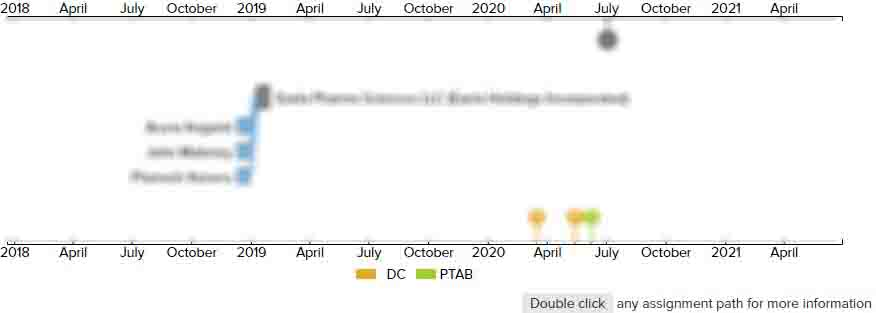# 2D/3D line rendering using 3D rasterization algorithms

• US 20060132495A1
• Filed: 12/21/2004
• Published: 06/22/2006
• Est. Priority Date: 12/21/2004
• Status: Active Grant
##### First Claim
Patent Images

1. A computer readable media embodying a method to compile a set of display points that define a two dimensional representation of a straight line graphic object using three dimensional rasterization algorithms, the method comprising:

• receiving data that defines three vertices (x0, y0, K0), (x1, y1, K1), and (x2, y2, K2) of a triangle primitive, where attributes K2=(K0+K1)/2, where coordinates (x0, y0), (x1, y1), and (x2, y2) form an isosceles triangle primitive, and where coordinates (x0, y0) and (x1, y1) define a base of the isosceles triangle primitive; and

rendering the isosceles triangle primitive using three dimensional rasterization algorithms to obtain the set of display points that define a two dimensional representation of the base of the isosceles triangle primitive.

• ##### 0 Assignments
Timeline View
Assignment View×
×
×